# Why's my program slow?

Algorithmic Complexity and You

## Hello

These slides are online at:

http://talks.edunham.net/linuxfestnorthwest2015/complexity/

Note

• I was in Computer Science at OSU for 5 years
• Many hats have included software developer (Intel and OSL)

Before upper-division CS classes, I thought there was some kind of secret sauce and complexity was super scary

Afterwards it's clear how simple things are, so much that the core concepts can fit into an hour at a conference

The difficulty is in the details, and this stuff is still indescribably tough to implement at the compiler level, but understanding the high-level view can help you ask smart questions and better understand real-world code

### Why this talk?https://www.flickr.com/photos/chefranden/3183416126/

Note

before I took algorithms classes at OSU, I thought complexity was secret magic that only "real computer scientists" could understand... afterwards it looked like common sense

not everybody in the real world gets the academic side; not everyone in academia gets the real-world side; i'm here to teach you about both

I hope you'll get 3 things today:
• Convinced that you can be a Real Computer Scientist too
• Tools for comparing algorithms
• A better set of basic questions to ask when analyzing program performance

### Agenda

In Theory

• Motivations for complexity analysis
• Meaning of the notation
• Techniques for analyzing complexity
• Examples

In Practice

• What problem are you solving?
• Alternate Tools

## In Theory

Coursera:
Syllabi:http://commons.wikimedia.org/wiki/File:Swiss_Jungfrau_mountains.jpg

Note

scope of this talk will be small though available knowledge is vast

jumping into the graduate level stuff here would be like a talk on getting started with recreational hiking focusing on what to take for a month in the Alps, rather than focusing on what to pack for a picnic in a nearby park

## What's complexity?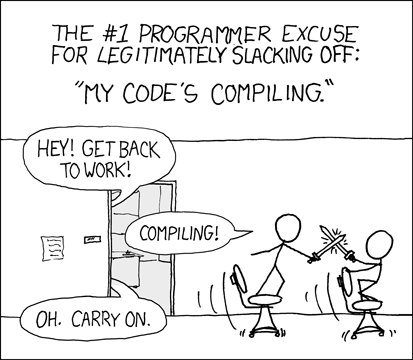https://imgs.xkcd.com/comics/compiling.png

Note

Why do they have time for a sword fight while the compiler runs?

There are two factors: The compiler has to do many operations (this scales with how big a program you compile) and each operation takes some time (this is something which can be optimized in the compiler)

### How Long it Takes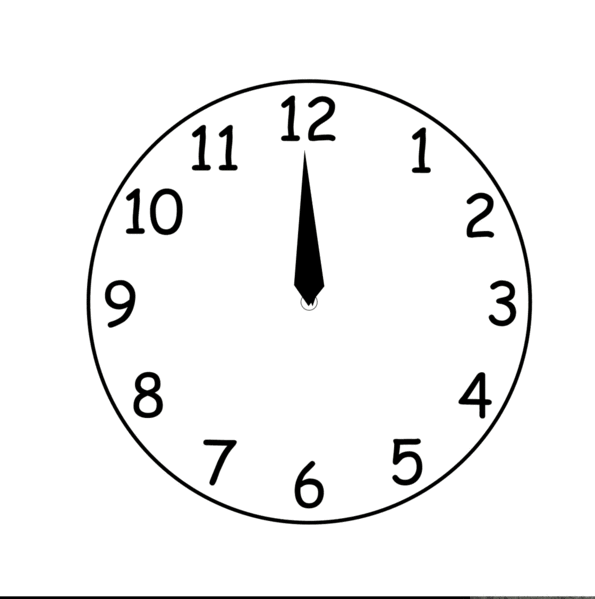http://commons.wikimedia.org/wiki/File:Analog_clock_animation.gif

Note

How long does your code take to run?

"About half an hour to run all the tests"?

NEXT: in terms of input size

### In terms of input sizehttps://projecteuler.net/

• Project Euler
• Solution fast on small input
• Very slow on large input

n is the size of the input.

N: /N/, quant.

1. A large and indeterminate number of objects: “There were N bugs in that

crock!” Also used in its original sense of a variable name: “This crock has N bugs, as N goes to infinity.”

http://www.catb.org/jargon/html/N/N.html

### Quantified in fancy notation

O(n)

(time in loop) * (times the loop runs) + (time outside of loop)

Note

Not like C the language; C like a constant amount of time

O(n)

Sound smarter? And write less? Win-Win!

Graph of why it's "usually ok to omit the constant" (more on that later)

### (Math Words)

For any monotonic functions f(n) and g(n) from the positive integers to the positive integers, we say that f(n) = O(g(n)) when there exist constants c > 0 and n > 0 such that:

```f(n) ≤ c * g(n), for all n ≥ n
```

### P vs NP

Note

How many of you really understood the P vs NP thing?

"NP problems are really 'hard', P problems are 'solvable'"

P is problems that can be SOLVED in polynomial time

NP is problems that can be VERIFIED in polynomial time

x^2 is polynomial; 2^x is exponential

Traveling salesman by brute force (shortest route between all cities) is O(n!)

http://en.wikipedia.org/wiki/Travelling_salesman_problem

### How do you find how many times it runs?https://www.flickr.com/photos/dalager/14110369/

Note

First find n

Simplify into psuedo-code till you just have loops

Examine them

(Basic test of fluency and understanding of your language of choice, like fizzbuzz)

There are also tools for this, which we'll get to later

### Find n

```needle = '4'
haystack = [2, 8, 23, 5, 4, 7, 42]

idx = 0
while haystack[idx] < len(haystack):
if haystack[idx] == needle:
print "Found it at index " + str(idx)
idx += 1
```

### Count the Loops

n is len(haystack)

```needle = '4'
haystack = [2, 8, 23, 5, 4, 7, 42]

idx = 0
while haystack[idx] < len(haystack):
if haystack[idx] == needle:
print "Found it at index " + str(idx)
idx += 1
```

### There's the complexity!

• loop once over n items
• Contents is constant time
• Complexity is O(n)

### Example: Finding Repeated Words

Same disclaimer as before.

```words = ['linux', 'washington', 'linux', 'festival']
idx = 0
while idx < len(words):
check = 0
while check < len(words):
if idx != check and words[check] == words[idx]:
print "found a repeated word!"
```

### First find n

```words = ['linux', 'washington', 'linux', 'festival']
idx = 0
while idx < len(words):
check = 0
while check < len(words):
if idx != check and words[check] == words[idx]:
print "found a repeated word!"
```

### Then count loops

n is len(words)

```words = ['linux', 'washington', 'linux', 'festival']
idx = 0
while idx < len(words):
check = 0
while check < len(words):
if idx != check and words[check] == words[idx]:
print "found a repeated word!"
```

### There's the complexity!

• Outer loop goes n times
• inner loop goes n times
• Inner loop runs every time the outer does
• Complexity is n*n, or n^2

### Tangent: If that was an interview...

```words = ['linux', 'washington', 'linux', 'festival']
idx = 0
while idx < len(words):
check = 0
while check < len(words):
if idx != check and words[check] == words[idx]:
print "found a repeated word!"
```

Note

faster to sort the list (sorts can go very fast) then traverse once, comparing each item to the previous

## What's the Difference?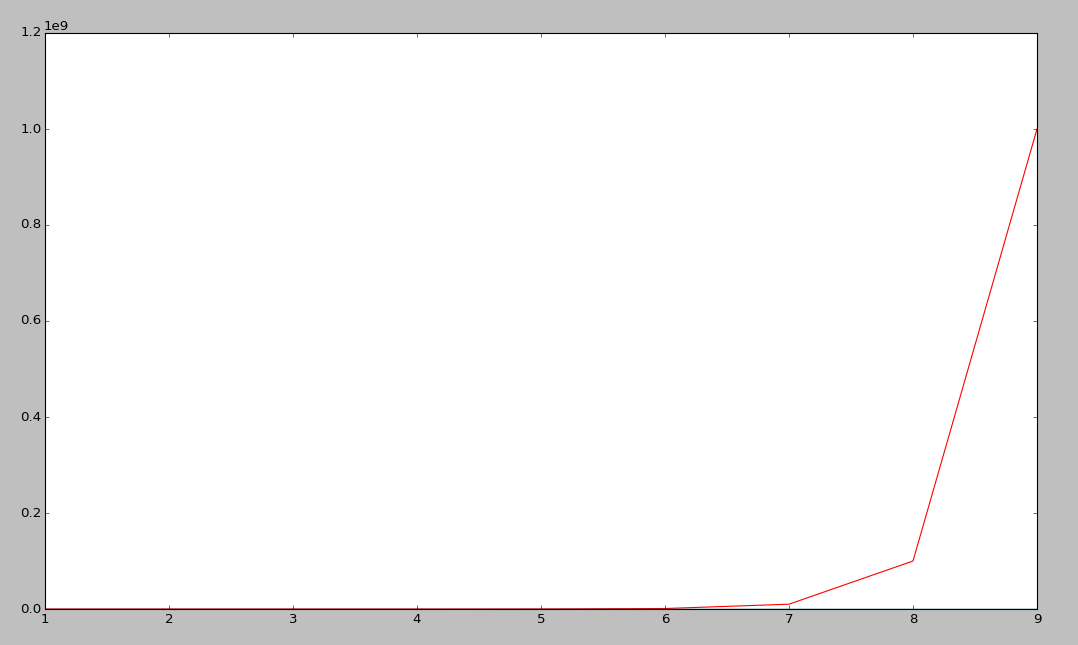Note

This graphs a bunch of complexities:

Exponential is red (constant raised to the n)

Linear is cyan

nlogn is green

logarithmic is blue

### Without exponential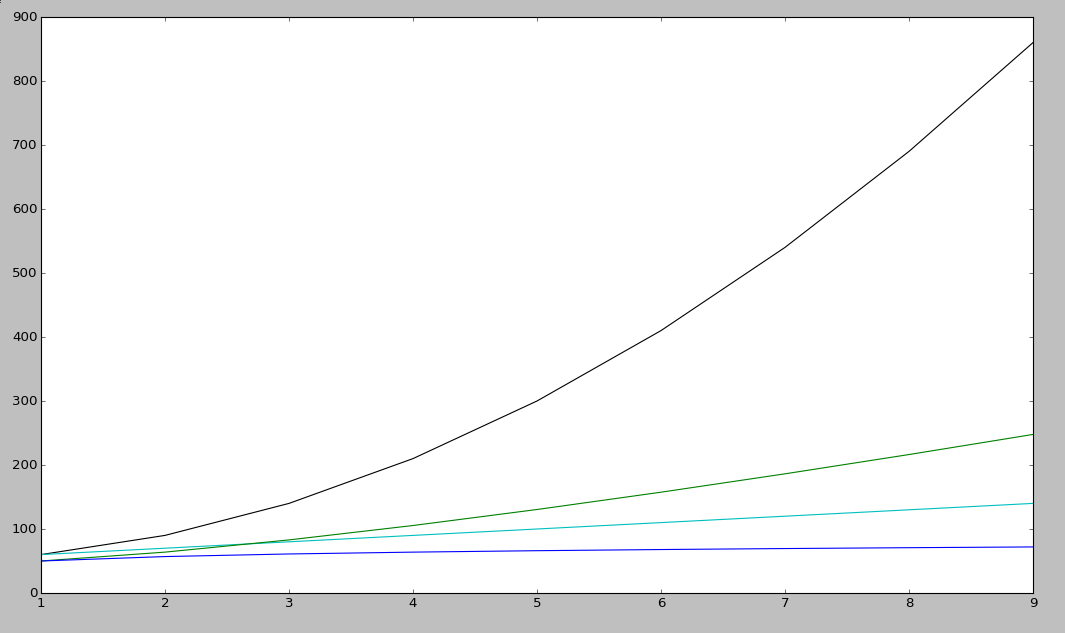Note

Linear is cyan

nlogn is green

logarithmic is blue

### Now you Try It

```def binary_search(l, value):
low = 0
high = len(l)-1
while low <= high:
mid = (low+high)//2
if l[mid] > value: high = mid-1
elif l[mid] < value: low = mid+1
else: return mid
return -1
```

### First find n

```def binary_search(l, value):
low = 0
high = len(l)-1
while low <= high:
mid = (low+high)//2
if l[mid] > value: high = mid-1
elif l[mid] < value: low = mid+1
else: return mid
return -1
```

### Count the loops

```def binary_search(l, value):
low = 0
high = len(l)-1
while low <= high:
mid = (low+high)//2
if l[mid] > value: high = mid-1
elif l[mid] < value: low = mid+1
else: return mid
return -1
```

### There's the complexity

Binary search is log(n)

"In mathematics, the logarithm of a number is the exponent to which another fixed value, the base, must be raised to produce that number."

### Some Details

big-oh is UPPER BOUND

big-omega is LOWER BOUND -- the program can never run faster than this

Big theta (not all programs will have this) is when upper and lower bounds match

### Amortized Complexity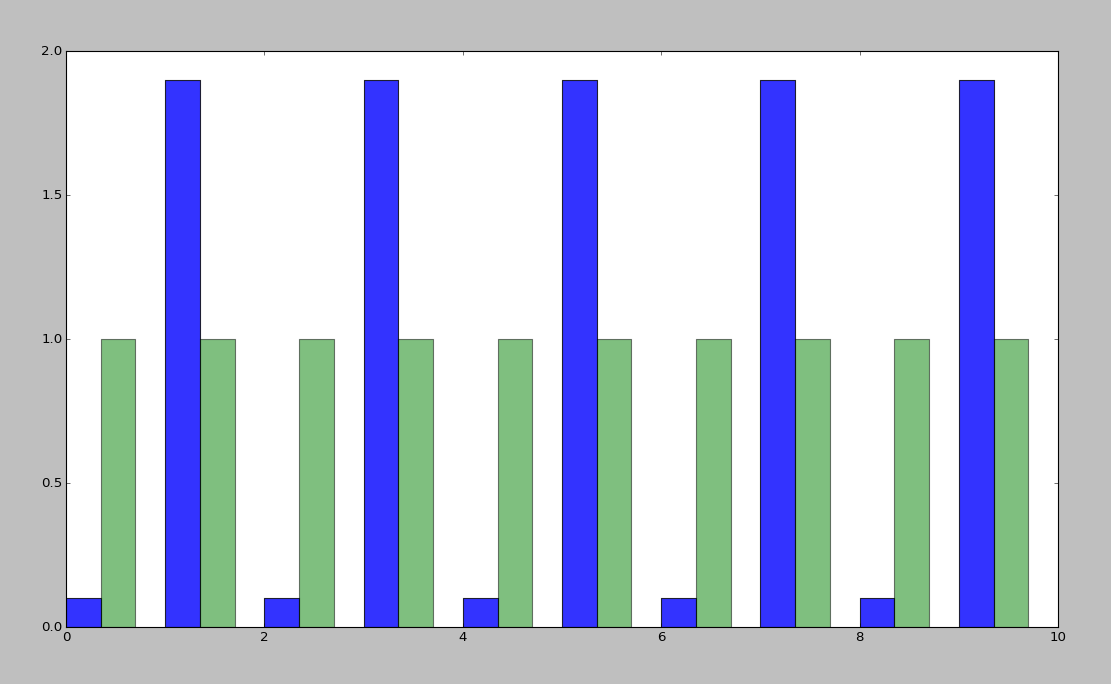If a slow operation is done infrequently, we can spread its cost over all the times it didn't happen...

```def binary_search(l, value, low = 0, high = -1):
if not l:
return -1
if(high == -1): high = len(l)-1
if low == high:
if l[low] == value: return low
else:
return -1
mid = (low+high)//2
if l[mid] > value:
return binary_search(l, value, low, mid-1)
elif l[mid] < value:
return binary_search(l, value, mid+1, high)
else: return mid
```

### Space Complexity

how much memory does it take?

In-place sorting vs sorting by copying the array

• Constant is the size of an item being sorted

Graphs of space complexity and show how they look quite a bit like time complexity

Note

TODO: sorting algos, in-place vs otherwise. example of very large arrays or very small memory, where this would actually matter

### Reversing an Array

copy elements:

```function reverse(a[0..n - 1])
allocate b[0..n - 1]
for i from 0 to n - 1
b[n − 1 − i] := a[i]
return b
```

vs in-place:

```function reverse_in_place(a[0..n-1])
for i from 0 to floor((n-2)/2)
tmp := a[i]
a[i] := a[n − 1 − i]
a[n − 1 − i] := tmp
```

## Feeling like a Real Computer Scientist yet?### That Constant

Constant times differ by several orders of magnitude.

• Processor memory vs L1 cache
• Cache vs RAM
• RAM vs hitting the disk
• Local disk vs across the datacenter
• Across the datacenter vs over wifi or a cell network
• Wifi or cell vs another continent

Note

Grace Hopper and the Nanoseconds

metaphor: going to the fridge vs going to the store vs going to the moon

## In The Real World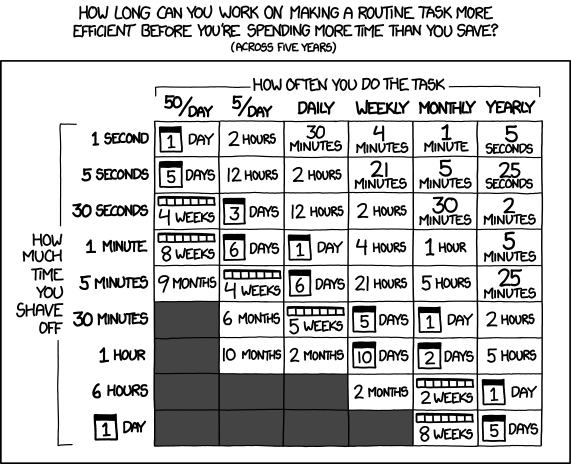Note

Approximately last 15mins?

same things apply to saving time in your algorithm

### Good Code

• Correct
• Efficient
• Simple
• Maintainable

Note

from cracking the coding interview, p. 56

### Is my program slow?

• Expected input size?

• How's it do in tests?
• (you are testing it, right?)
• How much of test suite time is setup/teardown?

• What will real-world conditions look like?
• and under heavy load? (CACHING CACHING CACHING)

Note

TODO: tools/frameworks for mocking heavy load on a program Worst case vs expected case

Slow to perform vs slow to write

### Is my program too slow?Note

Is speed the worst problem that it has right now?

What's the minimum that'll make your users happy?

What's the maximum past which your users won't notice improvements?

How long will it take the team to make the next big speedup...

• And would fixing any of the intermediat issues decrease that time substantially? (ie refactor to remove old cruft)

### Why is my program slow?

It's probably not how you structured your algorithm. Or you fix the obvious algorithmic stupidity and it's still bad.

Remember the orders of magnitude thing?

Note

• Profiling tools are your friends
• platform-agnostic -- how to get a graph of performance for various inputs
• language-specific profiling tools
• maybe it's slow in the real world because reality is different from your test cases
• Hard to see where an algorithm spends its time
• Working from a wrong guess about where the bottleneck is will waste yours

Note

"a slow program" could mean two things: code that's not performant, or code that takes forever to acutally get written. sometimes one is worse than the other.

### Algorithmic complexity in real code

• "the pros" can look at a section of code and tell you its best, worst, and average-case performance -- kind of like how we practiced earlier
• Recognizing patterns from having read a lot of other code

Note

• If the patterns aren't clear to you, write out what your code is doing

-- psuedo-code -- simplify it till all you have are bits that'll take constant time, and loops

### Expected Use Case

• Exponential time with a tiny constant vs. logarithmic with huge constant
• Input size?
• Space constraints?

Note

TODO: GRAPHS of high constant vs low constant, fast vs slow -- same graphs as before -- AGAIN, this is why context is critical

### Analysis Tools

Note

TODO: sort them * specific vs general * automated vs manual * language-specific vs platform-agnostic

Each language has its own

Python: Run Snake Run

C: GDB/gprof

### Instrumentation

http://en.wikipedia.org/wiki/Instrumentation_%28computer_programming%29http://commons.wikimedia.org/wiki/File:Pork_thermometer.jpg

### Call Profilershttp://en.wikipedia.org/wiki/File:Alt_Telefon.jpg

Note

Examine call stack, cprofile python in stdlib

https://docs.python.org/2/library/profile.html

### Testing

• Language or shell timing utilities
• Test on different sizes of input
• Write a test suite to catch preformance changes

### When to test?

• Always! (Continuous Integration)
• Deeper analysis for debugging, refactors

• How hard is it to introduce a complexity change?

### Recap

• Simplify the code
• Count how often it'll run for a given size of input
• Toss out the constants
Complexity isn't the entire picture
• That constant actually matters
• Premature optimization is bad for everyone
• Code that never gets finished was the slowest of all
Tools are great, in their place
• Instrumentation hooks into your code
• Profilers watch where it spends its time
• Test suite can check how long it takes on every change to find regressions

## Thanks!

These slides are online at:

http://talks.edunham.net/linuxfestnorthwest2015/complexity/